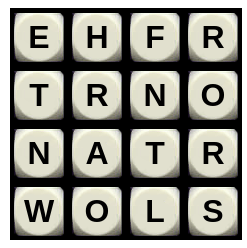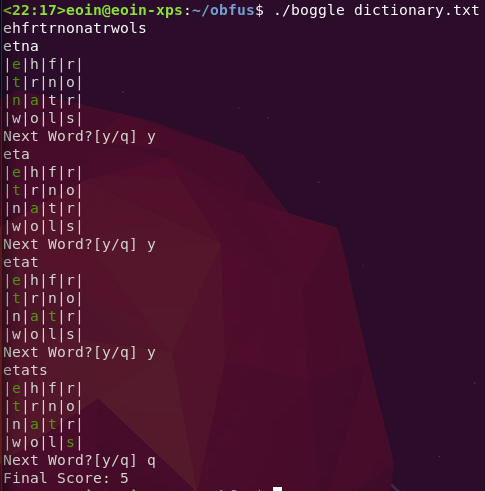# Vey.ie

Eoin Davey.
- MSc. Mathematics @ Universiteit van Amsterdam.
- Éireannach.

## Boggle.c

Have you ever wanted a program to play boggle for you, but haven’t been happy with how readable the available programs are?

Then I have good news for you, below you can find a boggle playing program with 0% readability but 100% boggle board shape

#include<stdio.h>
#include<stdlib.h>
#include<string.h>

char m[]="a$-??'" "$-$" "??'??'-??'$??'"""                                                          "??'(("
"())*+-\\34;6zI95("                                                         "?z\0"
"\x1B[3"     "2m\0"                                                         "\x1B["
"37m"      "\0Ja|"   "p$Skv;_}" "+uY$\0""_  *__,"   "90],b   ;v[4"     "sr,i;/****"
"38?M4     ^O><g:/ *(UkaA)WW=GJ€eV   4[=b'F?a2@9L/)LnW$g,HX7>[(%?G&pc*c;pE^p:X =$L'B[:763ckRmW"
"+B*;i<r@Kg*H-O2h BNZ08l1R?KYYGeV5* 7=!ogpmLAZYr4jZcP8 mkG]:4h>O&T&MW#WZj%17+/Ch  fGPQ@J:86T@RO-Y=5?"
"KJ]-U_VK7?YnSMK  nVMil39b*=[bD89GWRFo4CFY7C^$[(g6V.XbVeE/W_jm9]DreR?e-L8hH8T Ro0;<:N^ M#9_l^" "cGWKa3jVM>_o3C0 7G1N=B [email protected]?&*3 r!_fE=l U7Y*QMr =.PIF(- %HUi#P [P8Li'a]A,'K@Tdk$G"
"cn?'     DK5VNV1:Vn0^     #'c4'-On/,p,F     K%39RX8 L(,DH>V     ?+oZ^D &D/V. )_XV@5D/9BXfV^BH/!"
"_-AKB     rIbL+-d[8?W%k     (*:IrP,XJc0QH     TdgDl'^ .hASU)-     >RY=>C# J.3?dd c[OP?=&[C>nXgaF-n"
"A4Rr$+TLhi*[Y<Q'L9 (0cB6b>0=aNBl$    TUCnfQi ]WW#:FTe    #-h7,em 5;mP@- Qf^mh=[-"
"78*5CB6jA[2cGE?NE)6F[ALXl^28SiVajj-'ORD+pU,L)0gMn='T!?Q EV]D=ljT:;48'cEr0I9$Li0WQKbE=HnP+9f0" "n[JN4V@KQ5Ge;^gb)d 3!,o!!Le%oZ*ANK:!$hg4Y,^C9<7gEIo*dP  1/2%[TJbE6$:=2Zd5YBfT/@. 0@eJ!$(:d?;R12:d"
"PQ,-&=p'1DZAI&0!@   7:MR6ZR>:!b8ZEV   m9m:%!?hBXE__Tr9,   l#jBVQZCTm]a^e$Y!J:T::= -Y24V;[<%f06Z_" "-p670l]W6D+c=gLo (rBLGX6(Cl2 i4D,Z!@5/kMB5;3 Ndgo5aQiR<:VQcT1o7AH)fr #+D8i%G6CojH" "f95T>E Y^KGOc" "E%OM4 'Dc]_3A T&:/i9 ]#4eSP" ",E^LBQU 6V4)*EW@ rF.KE<Rd 7Bd'4D^" "Y@E*.n(H19UNml%U4 g&)7<A':o]bL<9aWP" "*Wd9Pk&#?$*[^5K    A5iNirl)V;b.YZ/"
"*",_,*__,u        ,b
;v[              4],sr,i;

typedef/**/     struct nd nd   ;struct nd{    int nd;nd*h[
26];}*r;is(     char*c,nd*r)   {if(*c&(1<<5   ))i=*c-*m,r
->h[i]=r->h[    i]?r->h[i]:    calloc(1,      sizeof(nd)),
is(c+1,r->h[    i]);r->nd=*c   &(1<<5)?r->    nd:1;}tr(int
x,int y,nd*r    ,int d){if(r   ->nd&&d>2){    sr+=d>7?11:m[
(1<<4)+d]&7;    if(pr(d))      return 1;}     for(int t=0;t

<8;++t){int     nx,ny;nx=1+x   -(m[t<<1]&3)   ,ny=1+y-(m[t
<<1|1]&3);if    (nx>=0&&ny>=   0&&nx<4&&ny<   4&&!v[nx][ny
]&&r->h[b[nx    ][ny]-*m]){v   [nx][ny]=1,u   [d]=b[nx][ny
];if(tr(nx,     ny,r->h[b[nx]  [ny]-*m],d+1)  )return 1;v[
nx][ny]=0,u[    d]='\0';}}     return 0;}pr(  d){puts(u);
for(i=0;i<16    ;++i)printf(   "%s%s%c%s|%s"  ,i&3?"":"|",v

[i>>2][i&3]?    m+38:"",b[i    >>2][i&3],v[   i>>2][i&3]?m
+44:"",(i+1)    &3?"":"\n");    __=m+50;      while(*__)
putchar(((*(    __++)-(1<<5)   )^(1<<2))+(1   <<5));scanf(
" %c",&_);     return _!='y';  }main(int ac,  char ** av){
FILE*d=fopen   (av,"r");r   =calloc(1,     sizeof(nd));
while(fgets(   u,90,d))is(u,r  );fclose(d);   memset(u,0,

sizeof(u));     for(i=0;i<16   ;++i)b[i>>2]   [i&3]=
getchar();      for(int t=0;    t<16;++t){    if(r->h[b[t>>
2][t&3]-*m])    {v[t>>2][t&3]   =1,u=b[t   >>2][t&3];if(
tr(t>>2,t&3,    r->h[b[t>>2][   t&3]-*m],1))  break; v[t>>2
][t&3]=0,u   ='\0';}}__=m+   24;while(*__  )putchar((*(__
++)-(1<<5))^    (sizeof(u))+(1  <<5));printf  ("%d\n",sr);}



### Compiling

This program must be compiled with the -trigraphs flag enabled

Ex: gcc -trigraphs -o boggle

This should be run in terminals supporting ANSI colour codes

(You will get a lot of warnings, this is the penance the code is paying for the sins involved in its creation)

### Usage

The syntax for running this program is ./boggle <dictionary file> The dictionary file must contain only lowercase latin characters The program will then accept the boggle board through stdin, input the board as a single line of 16 lowercase latin characters The program will then print the words on the board, one at a time, highlighting the required letters in green

### Example### The Algorithm

Before explaining the algorithm I’m assuming knowledge of graphs and tries

The thing to notice about a boggle board is that it is an implicit trie. The intuition for this is that chains of adjacent letters are exactly like paths through a tree structure. By interpreting the boggle board as a trie we can efficiently process it

The algorithm works as follows

1. Construct a trie from the given dictionary file
2. Use a depth first search approach to find the common paths of the dictionary trie and the the implicit boggle trie, ie. Traverse the boggle board depth first, only taking paths that match existing paths in the dictionary trie
3. For every full word reached, print the word and its path through the board

### The Obfuscation

The program above uses a lot of common obfuscation tactics, aided largely by my own unreadable style of programming. My personal favorite part is the string at the top that writes out “Boggle”, 90% of the string is randomly generated garbage, but the logic for moving on a 2d plane, the logic for scoring the game, and the logic for the hardcoded strings are all hidden in the string